﻿ 小麦哲伦云同步辐射信号与暗物质湮灭信号的研究

# 小麦哲伦云同步辐射信号与暗物质湮灭信号的研究Study on Synchrotron Radiation Signal and Dark Matter Annihilation Signal in Small Magellanic Cloud

Abstract: High-energy positrons and electrons lose energy in the magnetic field to produce synchrotron radia-tion signals. In irregular dwarf galaxies, both dark matter self-annihilation and cosmic rays produce high-energy positrons and electrons. In this paper, we review the synchrotron radiation signals in the Small Magellanic Cloud, an irregular dwarf galaxy in the neighborhood, and compare the con-straints on dark matter mass with and without cosmic ray contributions. We first assume that all the synchrotron radiation signals are generated by dark matter annihilation. Given the thermal relic annihilation cross section, the mass constraints on dark matter particles are limited by fitting observed data under the assumption of three different typical dark matter annihilation channels and we find is the best annihilation channel to fit the spectrum shape. Furthermore, we calculate the synchrotron radiation signal generated by cosmic rays through radio-infrared correlation, subtracting the contribution of this part of the detected flux and using the remaining part as the upper limit from the self-annihilation of dark matter. Taking into account annihilation channel, we give the corresponding dark matter mass under the two magnetic field assumptions by adjusting the dark matter mass to fit the data. When the contribution of cosmic rays is reasonably deducted, the upper mass of dark matter particles allowed is larger than that obtained without cosmic ray contribution subtracted at the same thermal annihilation cross-section.

1. 引言

2. 小麦哲伦云的射电能谱

2.1. 小麦哲伦云射电数据拟合

${S}_{\nu }={S}_{0}{\left(\frac{\nu }{{\nu }_{0}}\right)}^{-{\alpha }_{0}}$ (1)Table 1. Radio data from SMC at low frequenciesFigure 1. Spectral energy distribution of SMC at low frequencies

2.2. 宇宙线贡献的同步辐射

$\text{log}\frac{{L}_{\text{1}.\text{4},\text{GHz}}}{\text{W}\cdot {\text{Hz}}^{-\text{1}}}=1.032×log\frac{{L}_{24,obs}}{{L}_{\odot }}+11.642$ (2)

${S}_{CR}={S}_{1}{\left(\frac{\nu }{{\nu }_{1}}\right)}^{-{\alpha }_{1}}$ (3)

${\alpha }_{0}$ 在文献中也得到了广泛的探讨，我们假设它是0.8  。因此，我们可以得到宇宙线幂律分布为： ${S}_{CR}=\text{7}\text{.0}{\left(\nu /\text{1}.\text{4GHz}\right)}^{-0.8}$

3. 限制暗物质粒子属性

3.1. 源函数

 (3)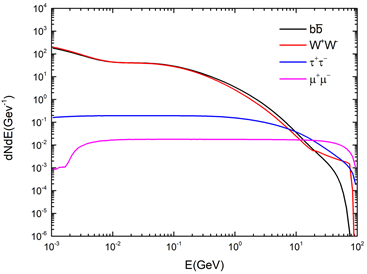Figure 2. Electron spectrums $\text{d}{N}_{e}/\text{d}E$ for different annihilation channels

${\rho }_{DM}\left(r\right)$ 是暗物质粒子密度，我们采用是NFW模型：

${\rho }_{NFW}\left(r\right)=\frac{{\rho }_{s}}{\left(\frac{r}{{r}_{s}}\right){\left[1+\left(\frac{r}{{r}_{s}}\right)\right]}^{2}}$ (4)

3.2. 暗物质湮灭同步辐射信号

${S}_{syn}\approx \frac{1}{{D}_{A}^{2}}\int \text{d}r{r}^{2}{j}_{syn}\left(\nu ,r\right)$ (5)

${j}_{syn}\left(\nu ,r\right)\text{}=2{\int }_{{m}_{e}}^{{M}_{\chi }}\text{d}E\frac{\text{d}{n}_{e}}{E}\left(E,r\right){P}_{syn}\text{}\left(\nu ,E,r\right)\text{}$ (6)

${P}_{syn}\left(\nu ,E,r\right)={\int }_{0}^{\text{π}}\text{d}\theta \frac{\mathrm{sin}\theta }{2}2\text{π}\sqrt{3}{r}_{0}{m}_{e}c{\nu }_{0}\mathrm{sin}\theta F\left(\frac{x}{\mathrm{sin}\theta }\right)$ (7)

$x\equiv \frac{2\nu \left(1+z\right){m}_{e}^{2}}{3{\nu }_{0}{E}^{2}}$ (8)

$F\left(s\right)\equiv s{\int }_{s}^{\infty }\text{d}\zeta {K}_{5/3}\left(\zeta \right)\approx 1.25{s}^{1/3}{e}^{-s}{\left[648+{s}^{2}\right]}^{1/12}$ (9)

$\frac{\text{d}{n}_{e}}{\text{d}E}\left(E,\stackrel{\to }{r}\right)=\frac{〈\sigma v〉{\rho }_{DM}^{2}\left(r\right)}{2b\left(E,r\right){m}_{\chi }{}^{2}}\underset{E}{\overset{{m}_{\chi }{c}^{2}}{\int }}{q}_{e}\left({E}^{\prime },r\right)\text{d}{E}^{\prime }$ (10)

${b}_{sync}=0.025{\left(\frac{B\left(\stackrel{\to }{r}\right)}{1\text{μG}}\right)}^{2}{\left(\frac{E}{1\text{GeV}}\right)}^{2}$ (11)

3.3. 限制暗物质粒子质量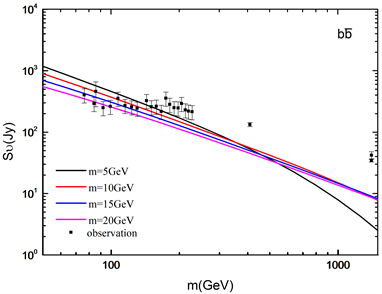Figure 3. The flux varies with frequency for the $b\stackrel{¯}{b}$ annihilation channel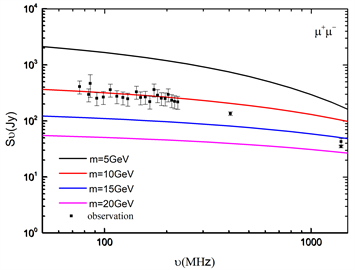Figure 4. The flux varies with frequency for the  annihilation channel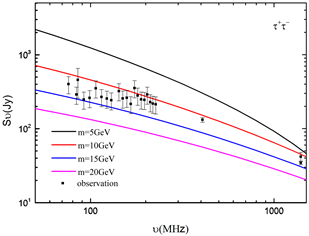Figure 5. The flux varies with frequency for the ${\tau }^{+}{\tau }^{-}$ annihilation channel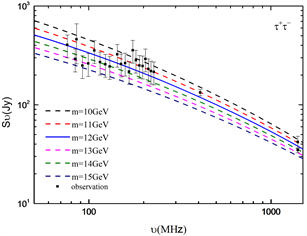Figure 6. The flux varies with frequency for the ${\tau }^{+}{\tau }^{-}$ annihilation channel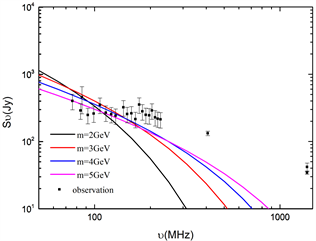Figure 7. The magnetic field is 1.7 mG and the observed data are fitted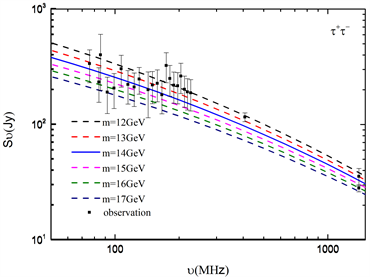Figure 8. After deducting cosmic rays, we fit the radio spectrum

4. 结论

 Feng, J.L. (2010) Dark Matter Candidates from Particle Physics and Methods of Detection. Annual Review of Astronomy and Astro-physics, 48, 495-545.
https://doi.org/10.1146/annurev-astro-082708-101659

 Bechtol, K., Drlica-Wagner, A., Balbinot, E., et al. (2015) Eight New Milky Way Companions Discovered in First-Year Dark Energy Survey Data. The Astrophysical Journal, 807, 50.

 Regis, M., Laura, R., Colafrancesco, S., Massardi, M., de Blok, W.J.G., Profumo, S. and Orford, N. (2015) Local Group dSph Radio Survey with ATCA-I: Observations and Background Sources. Monthly Notices of the Royal Astronomical Society, 448, 3731-3746.
https://doi.org/10.1093/mnras/stu2747

 Spekkens, K., Mason, B.S., Aguirre, J.E. and Nhan, B. (2013) A Deep Search for Extended Radio Continuum Emission from Dwarf Spheroidal Galaxies: Implications for Particle Dark MATTER. The Astrophysical Journal, 773, 61.
https://doi.org/10.1088/0004-637X/773/1/61

 Natarajan, A., Peterson, J.B., Voytek, T.C., Spekkens, K., Mason, B., Aguirre, J. and Willman, B. (2013) Bounds on Dark Matter Properties from Radio Observations of Ursa Major II Using the Green Bank Tele-scope. Physical Review D, 88, Article ID: 083535.
https://doi.org/10.1103/PhysRevD.88.083535

 For, B.-Q., Staveley-Smith, L., Hurley-Walker, N., et al. (2018) A Multifrequency Radio Continuum Study of the Magellanic Clouds—I. Overall Structure and Star Formation rates. Monthly Notices of the Royal Astronomical Society, 480, 2743-2756.

 Tasitsiomi, A., Gaskins, J. and Olinto, A.V. (2004) Gamma-Ray and Synchrotron Emission from Neutralino Annihilation in the Large Magellanic Cloud. Astroparticle Physics, 21, 637-650.
https://doi.org/10.1016/j.astropartphys.2004.04.004

 Mills, B.Y. (1959) Radio Frequency Radiation from External Galaxies. In: Flügge, S., Ed., Astrophysik IV: Sternsysteme/Astrophysics IV: Stellar Systems, Vol. 11/53, Springer, Berlin, Heidelberg, 239-274.
https://doi.org/10.1007/978-3-642-45932-0_6

 Lonsdale, C.J., et al. (2009) The Murchison Widefield Array: Design Over-view. Proceedings of the IEEE, 97, 1497-1506.

 Lawton, B., Gordon, K.D., Babler, B., et al. (2010) Spitzer Analysis of H II Region Complexes in the Magellanic Clouds: Determining a Suitable Monochromatic Obscured Star Formation Indicator. The As-trophysical Journal, 716, 453.
https://doi.org/10.1088/0004-637X/716/1/453

 Condon, J.J. (1992) Radio Emission from Normal Galaxies. Annual Review of Astronomy and Astrophysics, 30, 575-611.
https://doi.org/10.1146/annurev.astro.30.1.575

 Gondolo, P., Edsjö, J., Ullio, P., Bergström, L., Schelke, M. and Baltz, E.A. (2004) DarkSUSY: Computing Supersymmetric Dark Matter Properties Numerically. Journal of Cosmology and Astroparticle Physics, 2004, 008.
https://doi.org/10.1088/1475-7516/2004/07/008

 Bekki, K. and Stanimirović, S. (2009) The Total Mass and Dark Halo Properties of the Small Magellanic Cloud. Monthly Notices of the Royal Astronomical Society, 395, 342-350.
https://doi.org/10.1111/j.1365-2966.2009.14514.x

 McDaniel, A., Jeltema, T., Profumo, S. and Storm, E. (2017) Multiwa-velength Analysis of Dark Matter Annihilation and RX-DMFIT. Journal of Cosmology and Astroparticle Physics, 2017, 027.
https://doi.org/10.1088/1475-7516/2017/09/027

 Storm, E., Jeltema, T.E., Splettstoesser, M. and Profumo, S. (2017) Syn-chrotron Emission from Dark Matter Annihilation: Predictions for Constraints from Non-detections of Galaxy Clusters with New Radio Surveys. The Astrophysical Journal, 839, 33.
https://doi.org/10.3847/1538-4357/aa6748

Top